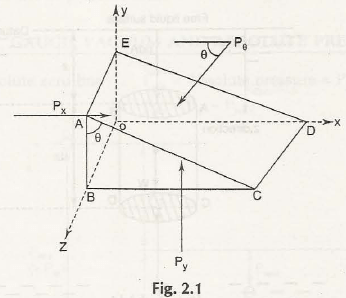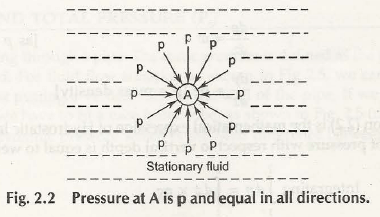Pascal’s law states that pressure exerted by the molecules at each and every point in an incompressible fluid is same. In other words, the intensity of pressure will be equal in all directions for static fluids.

Let us consider a fluid element at point P as shown in Fig. 2.1The pressure on the surface ABOE be Px

The pressure on the surface BCDO be Py

The pressure on the surface ACDE be PѲ

And,

AB = dy ­­

BC = dx

AE = dz

AC = ds

If the force on the surface ABOE be Px dydz

The force on the surface BCDO be Py dxdz

The force on the surface ACDE be PѲ dzds

According to Pascal’s law, fluid element is in stable equilibrium. So, we get-

x = 0

Px dydz – PѲ dzds cos  = 0

Or,

Px dydz – PѲ dydz = 0               (ds cos  = dy)

Px = PѲ

Now,

y = 0

Py dxdz – PѲ dzds sin  = 0

Or,

Py dxdz – PѲ dxdz = 0               (ds sin  = dx)

Py = PѲ

Hence, we can write it as-

Px = Py = PѲ

Or,

It shows that pressure is independent of angle of applied forces. It suggests that pressure is equal in all directions as shown in Fig. 2.2.In other words, it is cleared that the pressure exerted by the molecules is transmitted to all the points within the fluid when it is at rest. This shows the principle of Pascal’s law and it was established by the French Mathematician, Blaise Pascal.

Links of Next Mechanical Engineering Topics:-### Customer Reviews

My Homework Help
Rated 5.0 out of 5 based on 510 customer reviews at